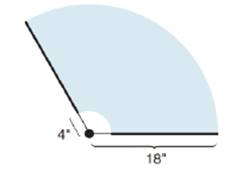Chapter 8.5, Problem 41E### Elementary Geometry for College St...

6th Edition
Daniel C. Alexander + 1 other
ISBN: 9781285195698

#### Solutions

Chapter
Section### Elementary Geometry for College St...

6th Edition
Daniel C. Alexander + 1 other
ISBN: 9781285195698
Textbook Problem
6 views

# A windshield wiper rotates through a 1200 angle as it cleans a windshield. From the point of rotation, the wiper blade begins at a distance of 4 in. and ends at a distance of 18 in. (The wiper blade is 14 in. in length.) Find the area cleaned by the wiper blade.To determine

To find:

The area cleaned by the wiper blade.

Explanation

Formula:

Area of sector:

If r is the radius of the circle, the area A of a sector whose arc has degree measure m is given by

A=m360×πr2

Calculation:

To find the area cleaned by wiper blade, we have to subtract the area of inner sector with radius 4 in. from the area of outer sector with radius 18 in.

Area cleaned by wiper blade =Aouter sector-Ainner sector

It is given that the wiper blade sweeps through an angle of 1200 as it cleans the windshield.

Hence the degree measure of arc is 1200.

Let’s find the area of outer sector.

From the diagram, it is clear that the radius r1 of outer sector is 18 in.

Let’s substitute the value of radius of outer sector and m=1200 to find the area of outer sector.

Asector=m360×πr12

Aouter sector=120360×π×182

Simplify: Aouter sector=13×π×324

Simplify: Aouter sector=108π in2.

Let’s substitute the value of π3.14

Aouter sector=108×3

### Still sussing out bartleby?

Check out a sample textbook solution.

See a sample solution

#### The Solution to Your Study Problems

Bartleby provides explanations to thousands of textbook problems written by our experts, many with advanced degrees!

Get Started

#### Find more solutions based on key concepts